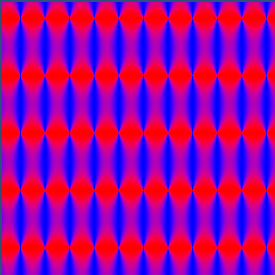# How to make a color graph in a SM3 Shader?

I am starting with shaders, and i would like to make a pattern generator (spectrography theory) displaying in unity. I would love a starter shader with color dependant on x and y axis. for example:

`red = sin(x * 5);`

``````blue = y*y;
``````

Thanks!

``````Shader "mShaders/mplasma3"
{
Properties
{
_Anim("Time", Float) = 0
_ResX("_ResX", Float) = 128
_ResY("_ResY", Float) = 128
}
{
Tags {Queue = Geometry}
Pass
{
CGPROGRAM
#pragma target 3.0
#pragma vertex vert
#pragma fragment frag
#include "UnityCG.cginc"

uniform float _ResX;
uniform float _ResY;
uniform float _Anim;

struct v2f {
float4 pos : POSITION;
float4 color : COLOR0;
float4 fragPos : COLOR1;
};

v2f vert (appdata_base v)
{
v2f o;
o.pos = mul (UNITY_MATRIX_MVP, v.vertex);
o.fragPos = o.pos;
o.color = float4 (1.0, 1.0, 1.0, 1);
return o;
}

half4 frag (v2f i) : COLOR
{
float4 outColor = i.color;
// main code, *original shader by: 'Plasma' by Viktor Korsun (2011)
float x = i.fragPos.x;
float y = i.fragPos.z;
float mov0 = x+y+cos(sin(_Anim)*2.)*100.+sin(x/100.)*1000.;
float mov1 = y / _ResY / 0.2 + _Anim;
float mov2 = x / _ResX / 0.2;
float c1 = abs(sin(mov1+_Anim)/2.+mov2/2.-mov1-mov2+_Anim);
float c2 = abs(sin(c1+sin(mov0/1000.+_Anim)+sin(y/40.+_Anim)+sin((x+y)/100.)*3.));
float c3 = abs(sin(c2+cos(mov1+mov2+c2)+cos(mov2)+sin(x/1000.)));
outColor.rgba = float4(c1,c2,c3,1);
return outColor;
}
ENDCG
}
}
FallBack "VertexLit"
}
``````

I’m just ramping up on CG programming this week, and am still at the stumbling/crawling stage. Here is a shader that I think gives you what you are looking for. Your calculation for blue is commented out in favor of something I found a bit more interesting.

``````Shader "Custom/Pattern" {
Properties {
_Mod1 ("Mod1", Float) = 20.0
_Mod2 ("Mod2", Float) = 10.0
}

Pass {
CGPROGRAM

#pragma exclude_renderers gles
// pragmas
#pragma fragment frag

uniform float _Mod1;
uniform float _Mod2;

//user defined variables
struct input {
float2 texcoord : TEXCOORD0;
};

// fragment function
float4 frag(input i) : COLOR {
float4 clr = float4(0,0,0,0);
clr.r =  (sin(i.texcoord.x * _Mod1) + 1.0) / 2.0 ;
clr.b =  (cos(i.texcoord.y * _Mod2) + 1.0) / 2.0 ;

//clr.b = i.texcoord.y*i.texcoord.y;
return normalize(clr);
}
ENDCG
}
}

fallback "Diffuse"
}
``````

With Mod values of 70 and 30 you get this:

##Take a look at stdlib here for CG functions:

http://http.developer.nvidia.com/Cg/index.html

I asked on the shaderlab forum and i have an answer… apparently i had to have a v2f vertex thing happening in the CG code, so here is some code that can graph things with many instructions. it can be handy for tron type worlds etc.

``````Shader "Custom/Pattern" {
Properties {
v1 ("Mod1", Float) = 300.0
v2 ("Mod2", Float) = 1.0
v3 ("Mod3", Float) = 1.0
}

Pass {
CGPROGRAM

#pragma vertex vert
#pragma fragment frag
#pragma target 3.0
#pragma exclude_renderers gles
#include "UnityCG.cginc"

uniform half v1;
uniform half v2;
uniform half v3;

//user defined variables
struct input {
float2 texcoord : TEXCOORD0;
};
struct v2f {
float4 pos : SV_POSITION;
float3 color : COLOR0;
float2 texcoord : TEXCOORD0;
};

v2f vert (appdata_base v)
{
v2f o;
o.pos = mul (UNITY_MATRIX_MVP, v.vertex);
o.color = v.normal * 0.5 + 0.5;
o.texcoord = v.texcoord;
return o;
}
// fragment function
float4 frag(input i) : COLOR {

half4 clr = half4(0.0,.0,.0,0.0);

half2 xy0 = i.texcoord + half2(-0.5, -0.5);
half c0 = length(xy0); //sqrt of xx+yy, polar coordinate radius math
clr.b=  (sin(c0 * v1)  ) ;

if (abs(clr.b) < .3){clr.b =1.0;}else{clr.b=0.0;}

//clr.g = clr.b ;

return normalize(clr);
}
ENDCG
}
}

fallback "Diffuse"
}
``````